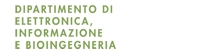Fabio Dercole

## Systems theory (nonlinear dynamics)

Milano Leonardo, 1st-half-sem. of the 1st-semester (5 credits)

News

• Starting with a.y. 2016/17, the course is scheduled in the full semester (not anymore in the first half).

General info

• Aims and learning outcomes
The course illustrates methods for the theoretical and numerical analysis of nonlinear dynamical systems and their application to case studies in the field of automation engineering. The focus is on the use of nonlinear systems theory, in particular bifurcation analysis, to classify the behaviours of the system and to understand the critical transitions occurring when some model or control parameters are varied. To this aim, the main bifurcations occurring in nonlinear systems are discussed in detail and illustrated first on simple examples and then, with the help of tools for numerical analysis, on case studies. A few bifurcations occurring in non-smooth systems (e.g., systems with impacts or switches) are also considered.

• Course organization
The course is organized in: lectures (30h), the theoretical notions are presented and illustrated with the help of simple examples and exercises; practicum (4h), further exercises for exam preparation; seminars (12h), case studies and numerical tools are introduced and discussed; computer labs (6h), standard software for numerical bifurcation analysis is used for analyzing examples and case studies.

• Final assessment
The final assessment consists in a written exam. A few extra points (to be added to the exam grade) can be obtained with an (optional) homework. The exam and homework can be replaced by a specific project to be discussed with the instructor.

• Course follow-up
The course is followed (in the second half of the semester) by the course Complessità nei sistemi e nelle reti (5 credits), taught (in Italian) by Prof. C. Piccardi. The two courses are highly integrated, in topic and style. Specifically, the second course extends the complexity of nonlinear phenomena from isolated systems to networks.

Syllabus and material

Lectures

Seminars

Computer labs

To be held in the lecture rooms. Students are invited to bring their own laptops with the proper numerical tools installed.

Numerical tools

Other material

• A book chapter:
• F. Dercole, S. Rinaldi, Dynamical Systems and Their Bifurcations, in Advanced Methods of Biomedical Signal Processing, eds. S. Cerutti, C. Marchesi, pp. 291–325, IEEE-Wiley Press, New York, NY, 2011 1994

• Two introductive books:
• S.H. Strogatz, Nonlinear Dynamics and Chaos, Addison-Wesley, 1994
Perhaps the best introductive textbook on nonlinear phenomena, rich in cross-disciplinary examples, with an affordable level of mathematical detail. The focus is on modeling and analysis of dynamical systems in both continuous and discrete time. Bifurcations and chaos are treated more phenomenologically than mathematically.

K. T. Alligood, T.D. Sauer, J.A. Yorke, Chaos: An Introduction to Dynamical Systems, Springer, 1996
It is a comprehensive introductive textbook, rich in cross-disciplinary examples and lab applications. Bifurcations are treated only marginally, while more emphasis and mathematical detail are put on chaos.

• S. Wiggins, Introduction to Applied Nonlinear Dynamical Systems and Chaos, Springer,1996
It is a comprehensive textbook on nonlinear dynamics for students seeking a rigorous mathematical formalism.

J. Guckenheimer, P. Holmes, Nonlinear Oscillations, Dynamical Systems and Bifurcations of Vector Fields, Springer, 1997 (5th ed.)
It is the historical “bibble” on nonlinear dynamical systems (first ed. 1983). High mathematical level.

Yu.A. Kuznetsov, Elements of Applied Bifurcation Theory, Springer, 2004 (3rd ed.)
It is the standard reference for bifurcation theory. Rather high mathematical level but readable with undergraduate notions of calculus and complex analysis.

Yu.A. Kuznetsov, O. Diekmann, W.-J. Beyn, Lecture notes of the master course Dynamical systems generated by ODEs and maps, University of Utrecht, The Netherlands
It is basically the preprint of a forthcoming introductory book. Still high mathematical level but readable with undergraduate notions of calculus and complex analysis.

• Scientific journals:
• Though many field-oriented scientific journals occasionally publish contributions dealing with nonlinear dynamical models, bifurcations, and chaos, a few interdisciplinary journals are explicitly dedicated to nonlinear phenomena. The most important are

International Journal of Bifurcation and Chaos (World Scientific)

Chaos (American Institute of Physics)

Chaos, Solitons and Fractals (Elsevier)

Physica D: Nonlinear Phenomena (Elsevier)

SIAM Journal on Applied Dynamical Systems (Society for Industrial and Applied Mathematics)

Homework

The homeworks consists in a numerical exercise assigned by the instructor to the class (not available online). The homework must be given in (in printed copy) by the day of the first exam. It gives at most 3 points to be added to the grade of the exam. The obtained score is valid for the entire academic year.

Top

Student hours

By appointment to be asked by e-mail to the instructor.

Written by Fabio Dercole